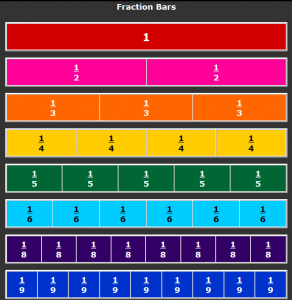# Teaching 4.NF.A.1- Equivalent Fractions using Visual Fraction Models

##Number & Operations – Fractions – 4th Grade

#### Extend understanding of fraction equivalence and ordering.

CCSS.Math.Content.4.NF.A.1
Explain why a fraction a/b is equivalent to a fraction (n × a)/(n × b) by using visual fraction models, with attention to how the number and size of the parts differ even though the two fractions themselves are the same size. Use this principle to recognize and generate equivalent fractions.

Teacher Notes
The foundation of fractions can only be built through visualizing fractions.  Students must be given many opportunities to manipulate fractions.  This can be done with fraction strips, by drawing fractions, using Interactive Fraction Bars on the computer or with any hands-on fraction manipulatives.  Students need to see that fractions with different denominators can be used to show the same quantities (this will be used later when finding least common denominator and greatest common factor).

Student Knowledge Goals

I can use visual fraction models appropriately.
I know when a denominator increases, the number of pieces it is divided into increases and the size of each piece decreases.
I know two fractions can be equivalent even though the numerators and denominators are different numerals.
I can explain how two fractions can be equivalent when the number of items in the sets they are describing is different.
I can determine when two fractions are equivalent.
I can explain and illustrate why fractions are equivalent or not equivalent.
I can generate equivalent fractions for a given fraction.

Vocabulary

compare
denominator
equivalent
factors
fraction
numerator

Lessons
Equivalent Fractions – Includes a visual for Smart Board and a video lesson
Fraction Bars – Equivalent fractions for Smart Board Viewing
*The Engage New York lessons starts with Decomposing Fractions (4.NF.B.3) and then builds into fraction equivalency and comparing.  Below is the order they suggest teaching.
Engage NY Module 5 A-1 – Decompose fractions as a sum of unit fractions using tape diagrams.
Engage NY Module 5 A-2 – Decompose fractions as a sum of unit fractions using tape diagrams.
Engage NY Module 5 A-3 – Decompose non-unit fractions and represent them as a whole number times a unit fraction using tape diagrams.
Engage NY Module 5 A-4 – Decompose fractions into sums of smaller unit fractions using tape diagrams.
Engage NY Module 5 A-5 – Decompose unit fractions using area models to show equivalence.
Engage NY Module 5 A-6 – Decompose fractions using area models to show equivalence.
Engage NY Module 5 B-7 – Use the area model and multiplication to show the equivalence of two fractions.
Engage NY Module 5 B-8 –  Use the area model and multiplication to show the equivalence of two fractions.
Engage NY Module 5 B-9 – Use the area model and division to show the equivalence of two fractions.
Engage NY Module 5 B-10 – Use the area model and multiplication to show the equivalence of two fractions.
Engage NY Module 5 B-11 – Explain fraction equivalence using a tape diagram and the number line, and relate that to the use of multiplication and division.

Student Video Lessons
Learn Zillion – Understand and explain equivalent fractions using visual models
Study Jams – Fraction Introduction (reteach/activate prior knowledge)
Study Jams – Equivalent Fractions
Virtual Nerd – 4.NF.A.1

Online Problems, Games, and Assessments
Khan Academy – Questions and Video Lessons
Equivalent Fractions
Patterns of  Equivalent Fractions
Interactive Fraction Bars